Home Practice
For learners and parents For teachers and schools
Textbooks
Full catalogue
Pricing SupportLog in

We think you are located in United States. Is this correct?

# Chapter 7: Measurement

• Use paper or cardboard for the net of solids to help learners see the different heights, particularly perpendicular and slanted heights.
• Units are compulsory when working with real life contexts.
• Sketches are valuable and important tools.
• Rounding off should only be done in the last step and level of accuracy should be relevant to the context.

This chapter is a revision of perimeters and areas of two dimensional objects and volumes of three dimensional objects. We also examine different combinations of geometric objects and calculate areas and volumes in a variety of real-life contexts.

## 7.1 Area of a polygon (EMBHV)

 Square$$\text{Area}={s}^{2}$$ Rectangle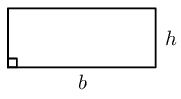$$\text{Area}=b\times h$$ Triangle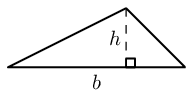$$\text{Area}=\frac{1}{2}b\times h$$
 Trapezium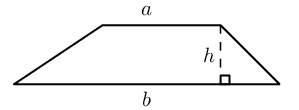$$\text{Area}=\frac{1}{2}\left(a+b\right)\times h$$ Parallelogram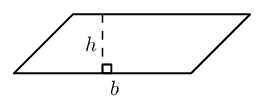$$\text{Area}=b\times h$$ Circle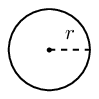$$\text{Area}=\pi {r}^{2}$$ $$\left(\text{Circumference}=2\pi r\right)$$
temp text

## Worked example 1: Finding the area of a polygon

$$ABCD$$ is a parallelogram with $$DC = \text{15}\text{ cm}$$, $$h = \text{8}\text{ cm}$$ and $$BF = \text{9}\text{ cm}$$.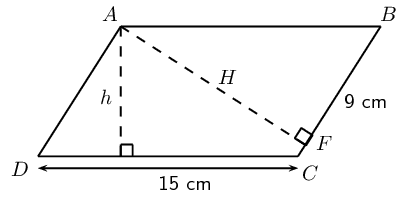Calculate:

1. the area of $$ABCD$$
2. the perimeter of $$ABCD$$

### Determine the area

The area of a parallelogram $$ABCD =$$ base $$\times$$ height:

\begin{align*} \text{Area} &= \text{15} \times \text{8} \\ &= \text{120}\text{ cm$^{2}$} \end{align*}

### Determine the perimeter

The perimeter of a parallelogram $$ABCD = 2DC + 2BC$$.

To find the length of $$BC$$, we use $$AF \perp BC$$ and the theorem of Pythagoras.

\begin{align*} \text{In } \triangle \text{ ABF:} \quad AF^2 &= AB^2 - BF^2 \\ &= \text{15}^2 - \text{9}^2 \\ &= \text{144} \\ \therefore AF &= \text{12}\text{ cm} \end{align*}\begin{align*} \text{Area} ABCD &= BC \ \times AF \\ \text{120} &= BC \times \text{12} \\ \therefore BC &= \text{10}\text{ cm} \end{align*}\begin{align*} \therefore \text{Perimeter} ABCD &=2(\text{15})+2(\text{10}) \\ &= \text{50}\text{ cm} \end{align*}

## Area of a polygon

Textbook Exercise 7.1

Vuyo and Banele are having a competition to see who can build the best kite using balsa wood (a lightweight wood) and paper. Vuyo decides to make his kite with one diagonal $$\text{1}$$ $$\text{m}$$ long and the other diagonal $$\text{60}$$ $$\text{cm}$$ long. The intersection of the two diagonals cuts the longer diagonal in the ratio $$\text{1}:\text{3}$$.

Banele also uses diagonals of length $$\text{60}$$ $$\text{cm}$$ and $$\text{1}$$ $$\text{m}$$, but he designs his kite to be rhombus-shaped.

Draw a sketch of Vuyo's kite and write down all the known measurements.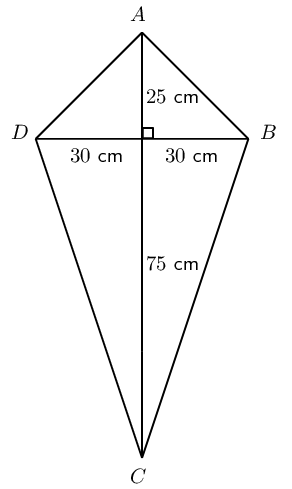Determine how much balsa wood Vuyo will need to build the outside frame of the kite (give answer correct to the nearest cm).
\begin{align*} AD &= AB \\ &= \sqrt{30^2+25^2} \quad (\text {Pythagoras}) \\ AD&=\text{39}\text{ cm} \\ DC&=BC \\ &=\sqrt{30^2+75^2} \quad (\text {Pythagoras}) \\ &=\text{81}\text{ cm} \\ \therefore \text{Balsa wood: } &= 2(81+39)\\ &=\text{240}\text{ cm} \end{align*}
Calculate how much paper he will need to cover the frame of the kite.
\begin{align*} \text{Area } &= 60 \times 100 \\ &= \text{6 000}\text{ cm$^{2}$}\\ &=\text{0,6}\text{ m$^{2}$} \end{align*}
Draw a sketch of Banele's kite and write down all the known measurements.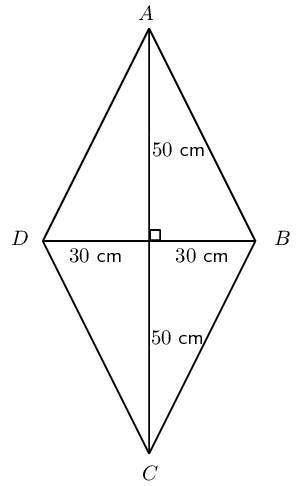Determine how much wood and paper Banele will need for his kite.
\begin{align*} \text{Side length} &= \sqrt{50^2+30^2} \\ &=\text{58,3}\text{ cm}\\ \therefore \text{Wood for frame } &= 4 \times \text{58,3}\text{ cm} \\ &= \text{233,2}\text{ cm}\\ \text{Area }&= 60 \times 100 \\ &= \text{6 000}\text{ cm$^{2}$}\\ &=\text{0,6}\text{ m$^{2}$} \end{align*}
Compare the two designs and comment on the similarities and differences. Which do you think is the better design? Motivate your answer.

Same amount of paper is required for both designs. Vuyo's designs uses more balsa wood.

$$O$$ is the centre of the bigger semi-circle with a radius of $$\text{10}$$ $$\text{units}$$. Two smaller semi-circles are inscribed into the bigger one, as shown on the diagram. Calculate the following (in terms of $$\pi$$):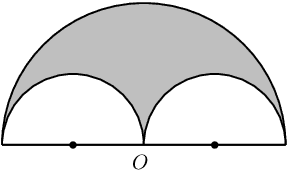The area of the shaded figure.
\begin{align*} \text{Area } &=\frac{1}{2}\pi(10)^2-2\left (\frac{1}{2}\pi(5)^2 \right )\\ &=50\pi-25\pi \\ &=25\pi \text{ units$^{2}$} \end{align*}
The perimeter enclosing the shaded area.
\begin{align*} \text{Perimeter }&=\frac{1}{2}(2\pi(10))+2\pi(5) \\ &=10\pi+10\pi \\ &=20\pi \text{ units$^{2}$} \end{align*}

Karen's engineering textbook is $$\text{30}$$ $$\text{cm}$$ long and $$\text{20}$$ $$\text{cm}$$ wide. She notices that the dimensions of her desk are in the same proportion as the dimensions of her textbook.

If the desk is $$\text{90}\text{ cm}$$ wide, calculate the area of the top of the desk.

\begin{align*} \text{Ratio } &= \frac{\text{table width}}{\text{book width}} \\ &=\frac{90}{20} \\ &=\text{4,5} \\ \therefore \text{Length of table } &= 30 \times \text{4,5} \text{ cm} \\ &=\text{135}\text{ cm}\\ \text{Area table} &= 135 \times 90 \\ &=\text{12 150}\text{ cm$^{2}$}\\ &=\text{1,2}\text{ m$^{2}$} \end{align*}

Karen uses some cardboard to cover each corner of her desk with an isosceles triangle, as shown in the diagram: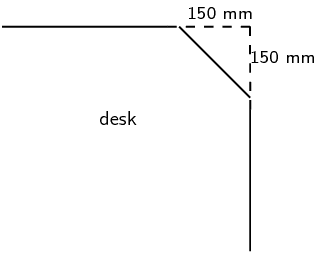Calculate the new perimeter and area of the visible part of the top of her desk.

\begin{align*} x^2&=15^2+15^2 \\ x&=\text{21,2}\text{ cm}\\ \text{New length}&=135-2(15) \\ &=\text{105}\text{ cm} \\ \text{New breadth}&=90-2(15) \\ &=\text{60}\text{ cm}\\ \text{New perimeter }&=2(105)+2(60)+4(\text{21,2})\\ &=\text{414,8}\text{ cm} \end{align*} \begin{align*} \text{Area cut off}&=2\times(15^2) \\ &=\text{450}\text{ m$^{2}$} \\ \text{New area } &= \text{12 150}\text{ cm$^{2}$} - \text{450}\text{ m$^{2}$} \\ &= \text{11 700}\text{ cm$^{2}$} \end{align*}
Use this new area to calculate the dimensions of a square desk with the same desk top area.
\begin{align*} s^2&= \text{11 700}\text{ cm$^{2}$} \\ \therefore s&= \text{108,2}\text{ cm}\\ \text{Square table of length} &\approx 108 \times 108 \text{ cm$^{2}$} \end{align*}